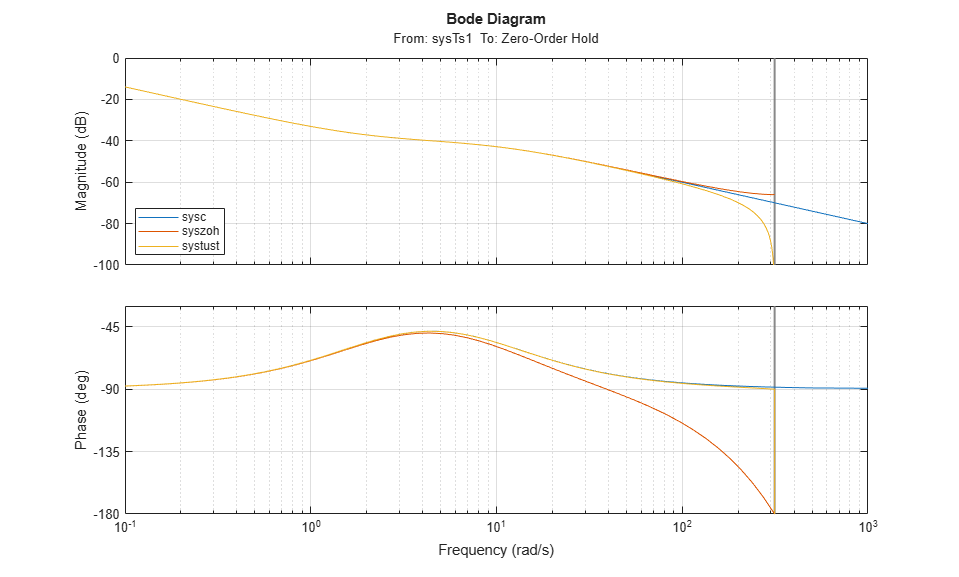# Linearize Models Using Different Rate Conversion Methods

This example shows how to specify the rate conversion method when linearizing a multirate Simulink® model. The choice of rate conversion methodology can affect the resulting linearized model. This example illustrates the extraction of a discrete linear time-invariant (LTI) model using two different rate conversion methods.

The `scdmrate` Simulink model contains five blocks with various sample times. All linear systems in this model are in zero-pole-gain format.

• `sysC` - Continuous-time linear time-invariant (LTI) system

• `Integrator` - Continuous-time integrator

• `sysTs1` - Discrete-time LTI system with a sample time of 0.01 seconds

• `sysTs2` - Discrete-time LTI system with a sample time of 0.025 seconds

• `Zero-Order Hold` - Block that samples the incoming signal at 0.01 seconds

```mdl = 'scdmrate'; open_system(mdl) ```In this example, you linearize the model between the output of the `sysTs1` block and the output of the `Zero-Order Hold` block. To compute an open-loop response, you add a loop opening at the output of the `Zero-Order Hold` block.

```io(1) = linio('scdmrate/sysTs1',1,'input'); io(2) = linio('scdmrate/Zero-Order Hold',1,'openoutput'); ```

Using these linearization points, the linearization effectively results in the linearization of the model `scdmrate_ol`.

```open_system('scdmrate_ol') ```When linearizing a model that contains both continuous and discrete signals, the software first converts the continuous signals to discrete signals, using a rate conversion method. To specify the rate conversion method, create a `linearizeOptions` object and set the `RateConversionMethod` property. The default rate conversion method is zero-order hold (`'zoh'`).

```opt = linearizeOptions; opt.RateConversionMethod ```
```ans = 'zoh' ```

Linearize the model using the default zero-order hold method. Since the linearization includes the `Zero-Order Hold` block, the sample time of the linearization is 0.01 seconds.

```syszoh = linearize(mdl,io,opt); ```

Change the rate conversion method to the Tustin (Bilinear transformation) method and linearize the model using this method. The sample time of the resulting model is also 0.01 seconds.

```opt.RateConversionMethod = 'tustin'; systust = linearize(mdl,io,opt); ```

You can also create a continuous-time linearized model by specifying the sample time as `0` in the `linearizeOptions` object. The rate conversion method creates a single discrete-time linearized model and then converts the discrete-time model to a continuous-time model.

```opt.SampleTime = 0; sysc = linearize(mdl,io,opt); ```

The Bode plots for the three linearizations show the effects of the two rate conversion methods. In this example, the Tustin rate conversion method gives the most accurate representation of the phase response of the continuous-time system and the zero-order hold gives the best match to the magnitude response.

```p = bodeoptions('cstprefs'); p.YLimMode = {'manual'}; p.YLim = {[-100 0];[-180 -30]}; p.Grid = 'on'; bodeplot(sysc,syszoh,systust,p); legend('sysc','syszoh','systust','Location','SouthWest'); ```Close the models.

```bdclose('scdmrate') bdclose('scdmrate_ol') ```